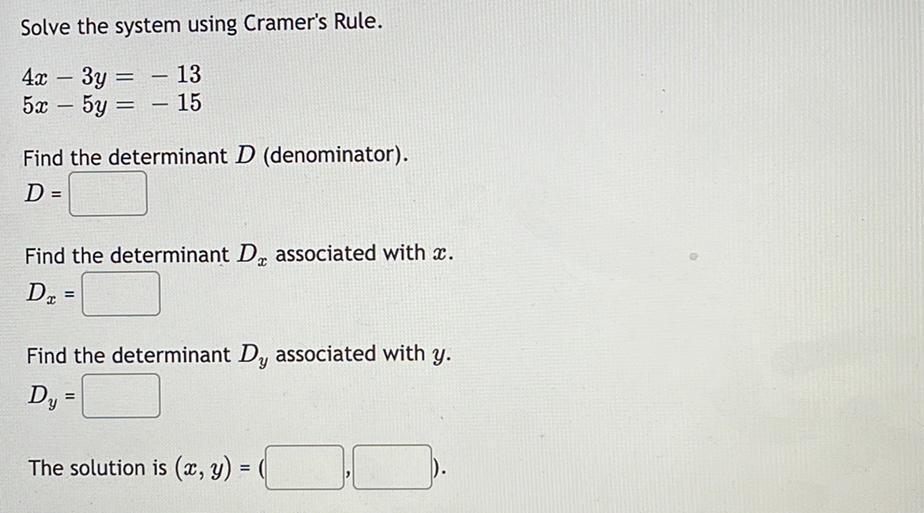Question:

# Solve the system using Cramer's Rule. 4x - 3y = -13 5x – 5y

Last updated: 7/26/2022Solve the system using Cramer's Rule. 4x - 3y = -13 5x – 5y = -15 Find the determinant D (denominator). D = Find the determinant Dₓ associated with x. Dₓ = Find the determinant Dᵧ associated with y. Dᵧ= The solution is (x, y) =( , ).